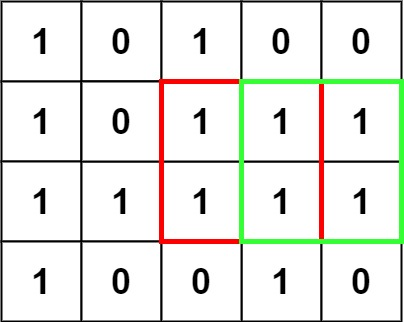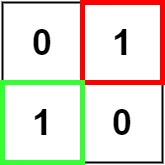| English | 简体中文 |

# 221. Maximal Square

## Description

Given an m x n binary matrix filled with 0's and 1's, find the largest square containing only 1's and return its area.

Example 1:Input: matrix = [["1","0","1","0","0"],["1","0","1","1","1"],["1","1","1","1","1"],["1","0","0","1","0"]]
Output: 4


Example 2:Input: matrix = [["0","1"],["1","0"]]
Output: 1


Example 3:

Input: matrix = [["0"]]
Output: 0


Constraints:

• m == matrix.length
• n == matrix[i].length
• 1 <= m, n <= 300
• matrix[i][j] is '0' or '1'.